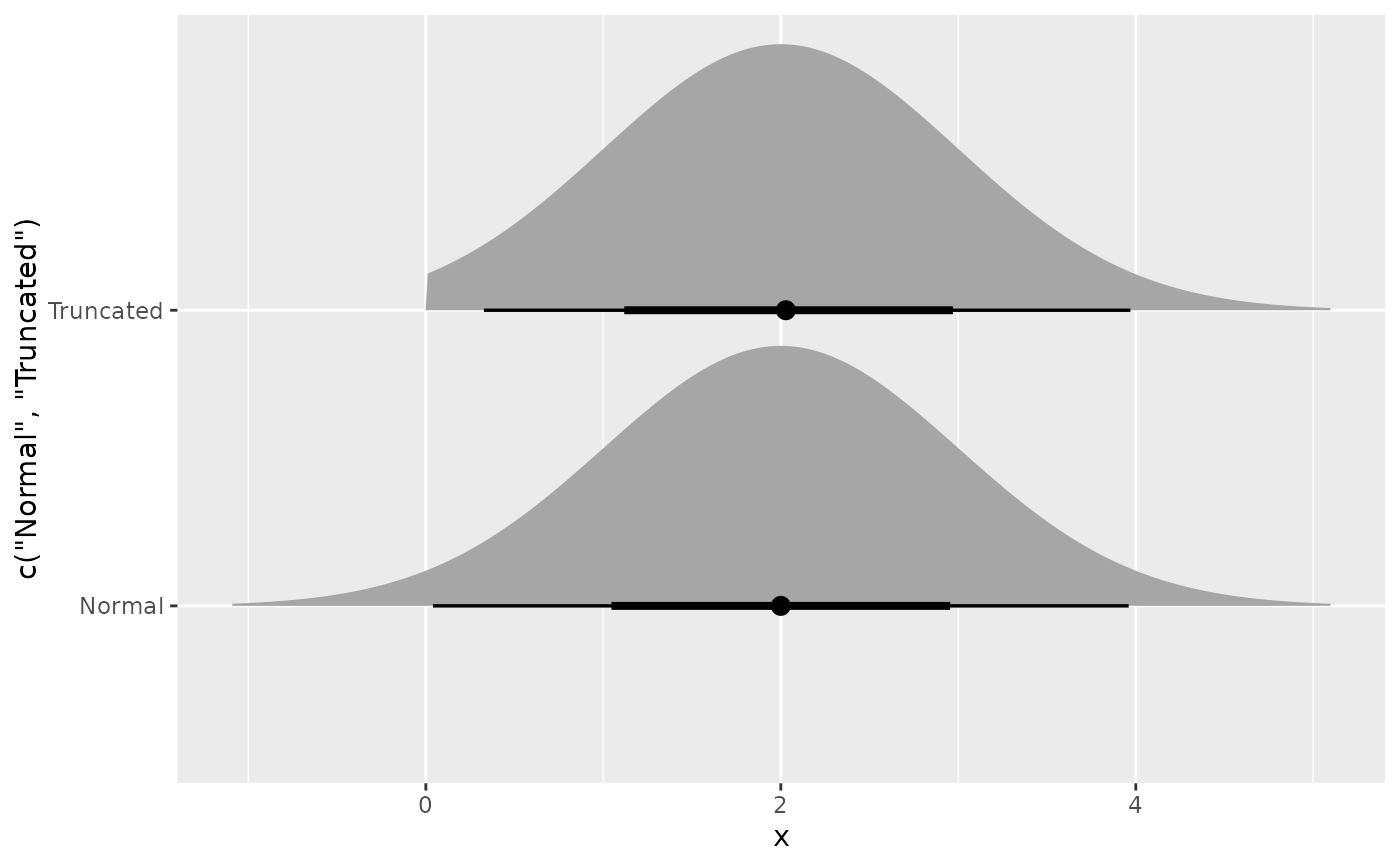dist_truncated(dist, lower = -Inf, upper = Inf)

## Arguments

dist The distribution(s) to truncate. The range of values to keep from a distribution.

## Details

Note that the samples are generated using inverse transform sampling, and the means and variances are estimated from samples.

## Examples

dist <- dist_truncated(dist_normal(2,1), lower = 0)

dist
#> <distribution>
#>  N(2, 1)[0,Inf]mean(dist)
#>  2.055248variance(dist)
#>  0.8396438
generate(dist, 10)
#> []
#>   0.9644266 0.8541601 2.2539323 3.5935605 1.0546828 3.2657823 0.9971509
#>   1.8244695 0.7747821 1.4118141
#>
density(dist, 2)
#>  0.4082296density(dist, 2, log = TRUE)
#>  -0.8959256
cdf(dist, 4)
#>  0.9767203
quantile(dist, 0.7)
#>  2.544133
if(requireNamespace("ggdist")) {
library(ggplot2)
ggplot() +
ggdist::stat_dist_halfeye(
aes(y = c("Normal", "Truncated"),
dist = c(dist_normal(2,1), dist_truncated(dist_normal(2,1), lower = 0)))
)
}
#> Loading required namespace: ggdist